Functions

This chapter discusses scalar functions and set functions (see Set Functions.)

Scalar Functions

A scalar function takes zero or more parameters and returns a single value. A scalar function can be used wherever an expression is allowed.

Scalar functions

ABS

ACOS

ASCII_CHAR

ASCII_CODE

ASIN

ATAN

ATAN2

BEGINS

BUILTIN.BEGINS_WORD

BUILTIN.MATCH_WORD

BUILTIN.UTC_TIMESTAMP

CHARACTER_LENGTH

CEILING

COS

COSH

COT

CURRENT_DATE

CURRENT_PROGRAM

CURRENT_USER

CURRENT VALUE

DAY

DAYOFMONTH

DAYOFWEEK

DAYOFYEAR

DEGREES

EXP

EXTRACT

FLOOR

HOUR

INDEX_CHAR

IRAND

LEFT

LN

LOCALTIME

LOCALTIMESTAMP

LOCATE

LOG10

LOWER

MINUTE

MOD

MONTH

NEXT VALUE

OCTET_LENGTH

OVERLAY

PASTE

POSITION

POWER

QUARTER

REGEXP_MATCH

REPEAT

REPLACE

RIGHT

ROUND

SECOND

SESSION_USER

SIGN

SIN

SINH

SOUNDEX

SQRT

SUBSTRING

TAIL

TAN

TANH

TRIM

TRUNCATE

UNICODE_CHAR

UNICODE_CODE

UPPER

USER

WEEK

YEAR

The following sections describe Mimer SQL’s scalar functions.

ABS

Returns the absolute value of the given numeric expression.

Syntax

Syntax for the ABS function: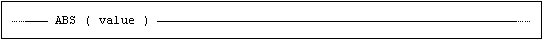value is a numeric or an interval value expression.

Description

The function returns the absolute value of value.

If the value of value is null, then the result of the function is null.

Example

SET INT_VAL = ABS(-15);  -- sets INT_VAL to 15

ACOS

Returns the arccosine for a numeric expression.

Syntax

Syntax for the ACOS function: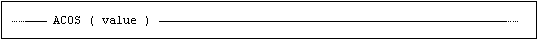value is a numeric value expression. The function handles values that are within the range of a double precision expression.

Rules

The functions returns the arccosine for the value expressed as radians. The data type for the result is double precision. Valid input values are in the range -1 to 1.

If the value of value is NULL, then the result of the function is NULL.

ASCII_CHAR

Returns the character that has the given ASCII code value. The given ASCII code value should be in the range 0-255.

Syntax

Syntax for the ASCII_CHAR function: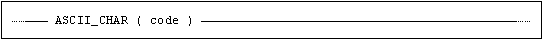code is a numeric expression representing an ASCII value.

Description

If the value of code is between 0 and 255, the function returns a single character value, i.e. CHAR(1), otherwise the function returns null. (For code values above 255, use the UNICODE_CHAR function instead. See UNICODE_CHAR.)

If the value of code is null, then the result of the function is null.

Example

SET CHR_VAL = ASCII_CHAR(65);  -- sets CHR_VAL to 'A'

ASCII_CODE

Returns the ASCII code value of the leftmost character in the given string expression, as an integer.

Syntax

Syntax for the ASCII_CODE function: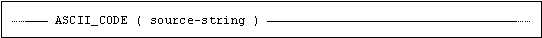source-string is a character or binary string expression.

Description

A single INTEGER value is returned, representing an ASCII code.

If the source-string contains more than one character, the ASCII code of the left-most octet is returned.

If the length of source-string is zero, then the result of the function is null.

If the value of source-string is null, then the result of the function is null.

Example

SET INT_VAL = ASCII_CODE('A'); -- sets INT_VAL to 65

ASIN

Returns the arcsine for a numeric expression.

Syntax

Syntax for the ASIN function: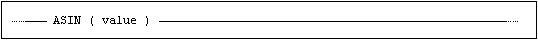value is a numeric value expression. The function handles values that are within the range of a double precision expression.

Rules

The functions returns the arcsine for the value expressed as radians. The data type for the result is double precision. Valid input values are in the range -1 to 1.

If the value of value is NULL, then the result of the function is NULL.

ATAN

Returns the arctangent for a numeric expression.

Syntax

Syntax for the ATAN function: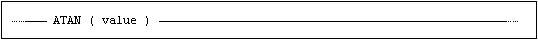value is a numeric value expression. The function handles values that are within the range of a double precision expression.

Rules

The functions returns the arctangent for the value expressed as radians. The data type for the result is double precision.

If the value of value is NULL, then the result of the function is NULL.

ATAN2

Returns the arctangent for the tangent between 2 numeric expressions.

Syntax

Syntax for the ATAN2 function: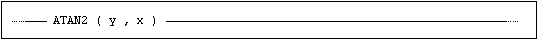value-1 and value-2 are numeric value expressions. The function handles values that are within the range of a double precision expression.

The ATAN2 function calculates the arctangent of the two parameters value-1 and value-2. It is similar to calculating the arctangent of value-2 / value-1, except that the signs of both arguments are used to determine the quadrant of the result. Effectively, this means that ATAN2(value-1, value-2) finds the counterclockwise angle in radians between the x-axis and the vector <value-2, value-1> in 2-dimensional Euclidean space.

Rules

Returns the angle, in radians, whose tangent is between the two given value expressions. The data type for the result is double precision.

If the value of value-1 or value-2 is NULL, then the result of the function is NULL.

BEGINS

Perform a “begins with” comparison.

Syntax

Syntax for the BEGINS function: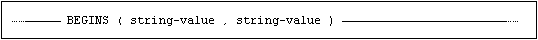Description

LIKE predicates, addressing the “begins with” functionality, are very common.

However, when a parameter marker is used for the LIKE pattern, the SQL compiler can not determine the LIKE pattern characteristics, and possible optimizations will not be applied. The built-in function BEGINS will overcome this issue.

Examples

BEGINS function

Is equivalent to

BEGINS(col,'AB')

col LIKE 'AB%'

BEGINS(col,?),
where ? contains 'XYZ'

col LIKE 'XYZ%'

BUILTIN.BEGINS_WORD

Returns a boolean denoting if there is a word in the search-string argument that begins with the word-part argument.

Syntax

Syntax for the BUILTIN.BEGINS_WORD function: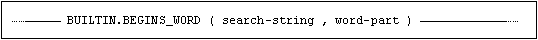Description

The search-string and the word-part arguments must both be character expressions (i.e. either CHARACTER/VARCHAR or NATIONAL CHARACTER/NVARCHAR.)

For this type of searches, the database will consider using a WORD_SEARCH index if appropriate. (See CREATE INDEX.)

If any of the arguments to the function is null the function returns null. The function will return true if there is a word in the search-string argument that begins with the characters in the word-part argument and false otherwise.

Trailing space characters in the <word-part> string are trimmed before the search operation. The <word-part> string may only contain characters that have the Unicode property "ID_Continue". For a detailed description, see https://www.unicode.org/reports/tr31.

Examples

SQL>set ? = builtin.begins_word('The quick brown fox jumps over', 'bro');

?

=====

TRUE

The following comparison will not match since the case of the word-part does not match.

SQL>set ? = builtin.begins_word('The quick brown fox jumps over','Bro');

?

=====

FALSE

It is possible to use collations for the arguments, for example to do a case insensitive search:

SQL>set ? = builtin.begins_word('The quick brown fox jumps',
SQL&'Bro' collate english_1);

?

====

TRUE

BUILTIN.MATCH_WORD

Returns a boolean denoting if there is a word in the search-string that matches the word argument.

Syntax

Syntax for the BUILTIN.MATCH_WORD function: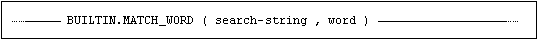Description

The search-string and the word arguments must both be character expressions, either character or national character.

For this type of searches, the database will consider using a WORD_SEARCH index if appropriate. (See CREATE INDEX.)

If any of the arguments to the function is null the function returns null. The function will return true if there is a word in the search-string argument that matches the word argument completely, and false otherwise.

Trailing space characters in the <word> string are trimmed before the match operation. The <word> string may only contain characters that have the Unicode property "ID_Continue". For a detailed description, see https://www.unicode.org/reports/tr31.

Examples

SQL>set ? = builtin.match_word('The quick brown fox jumped', 'bro');

?

=====

FALSE

SQL>set ? = builtin.match_word('The quick brown fox jumped', 'brown');

?

=====

TRUE

SQL>create index docind on ducuments (content for word_search);

SQL>select * from documents where builtin.match_word(content, 'Mimer');

BUILTIN.UTC_TIMESTAMP

Returns a timestamp denoting the current Coordinated Universal Time.

Syntax

Syntax for the BUILTIN.UTC_TIMESTAMP function: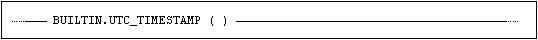Description

The result is the current Coordinated Universal Time as a timestamp value.

All references to BUILTIN.UTC_TIMESTAMP are effectively evaluated simultaneously from a single reading of the server clock. Thus the conditional expression BUILTIN.UTC_TIMESTAMP() = BUILTIN.UTC_TIMESTAMP() is guaranteed to always evaluate to true.

Examples

SQL>SELECT BUILTIN.UTC_TIMESTAMP() AS utcts FROM system.onerow;

utcts

=====

2012-10-30 14:55:22.643082

One row found

CREATE TABLE EVENTS(ID INTEGER PRIMARY KEY,

UTCTS TIMESTAMP);

INSERT INTO EVENTS(ID) VALUES (1, BUILTIN.UTC_TIMESTAMP());

UPDATE EVENTS

SET    ID = ID + 5, UTCTS = BUILTIN.UTC_TIMESTAMP()

WHERE  ID = 10;

CHARACTER_LENGTH

Returns the length of a string.

Syntax

Syntax for the CHARACTER_LENGTH (or CHAR_LENGTH) function: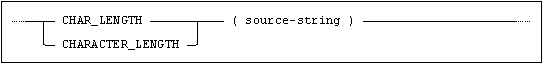source-string is a character or binary string expression.

Description

CHAR_LENGTH returns an INTEGER value.

If the data type of source-string is variable-length character or variable-length binary, then the result of CHAR_LENGTH is the same as the actual length of source-string.

If the data type of source-string is fixed-length character or fixed-length binary, then the result of CHAR_LENGTH is the same as the fixed-length of source-string.

If the value of source-string is null, then the result of the function is null.

Example

SET INT_VAL = CHAR_LENGTH('TEST STRING'); -- sets INT_VAL to 11

CEILING

Returns the smallest integer greater than or equal to a numeric expression.

Syntax

Syntax for the CEILING function: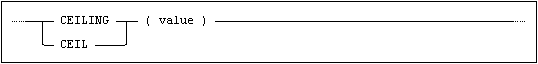value is a numeric value expression.

Description

The function returns the nearest integer value that is equal or higher to value.

If the value of value is null, then the result of the function is null.

The return data type is based on the input data type. For DECIMAL input, the return data type is integer.

Example

SET ? = CEILING(3.57);     -- returns 4

SET ? = CEILING(-3.57);    -- returns -3

SET ? = CEILING(1.2345e3); -- returns 1.235000000E+003

COS

Returns the cosine for a numeric expression.

Syntax

Syntax for the COS function: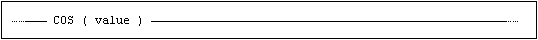value is a numeric value expression. The function handles values that are within the range of a double precision expression.

Rules

The functions returns the cosine for the values expressed as radians. The data type for the result is double precision.

If the value of value is NULL, then the result of the function is NULL.

COSH

Returns the for hyperbolic cosine a numeric expression.

Syntax

Syntax for the COSH function: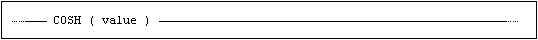value is a numeric value expression. The function handles values that are within the range of a double precision expression.

Rules

The functions returns the hyperbolic cosine for the values expressed as radians. The data type for the result is double precision.

If the value of value is NULL, then the result of the function is NULL.

COT

Returns the cotangent for a numeric expression.

Syntax

Syntax for the COT function: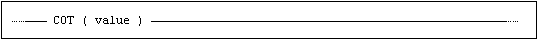value is a numeric value expression. The function handles values that are within the range of a double precision expression.

Rules

The function returns the cotangent for the value, expressed as radians. The data type for the result is double precision.

If the value of value is NULL, then the result of the function is NULL.

CURRENT_DATE

Returns a DATE value denoting the current date (i.e. today).

Syntax

Syntax for the CURRENT_DATE function: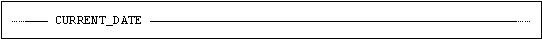Description

The result is the current date (i.e. today) as a DATE value.

All references to CURRENT_DATE are effectively evaluated simultaneously from a single reading of the server clock. Thus the conditional expression CURRENT_DATE = CURRENT_DATE is guaranteed to always evaluate to true.

The value of CURRENT_DATE will always be equal to the DATE portion of LOCALTIMESTAMP.

Example

UPDATE sometable SET usercnt = 13, updated = CURRENT_DATE;

CURRENT_PROGRAM

Returns the name of an entered program.

Syntax

Syntax for the CURRENT_PROGRAM function: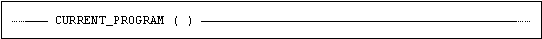Description

The function returns the value of the most recently entered program as nchar varying value with a maximum length of 128, with the collation SQL_IDENTIFIER.

If no program has been entered the result of the function is null.

Example

The following example returns the PROGRAM ident if entered, otherwise the session ident:

SET CHR_STR = COALESCE(CURRENT_PROGRAM(), SESSION_USER);

CURRENT_USER

Returns the name of the currently connected USER ident or the PROGRAM ident that is currently entered.

When used in a routine or trigger, it returns the name of the creator of the schema to which the routine or trigger belongs.

Syntax

Syntax for the CURRENT_USER function: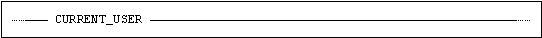Description

When used in a routine or trigger, the result is the name of the creator of the schema to which the routine or trigger belongs, otherwise the value is the name of the connected ident or the program that was entered.

The data type of the returned value is nchar varying with a maximum length of 128, with the collation SQL_IDENTIFIER.

Example

CREATE DOMAIN NAME AS NCHAR VARYING(128) collate SQL_IDENTIFIER DEFAULT CURRENT_USER;

CURRENT VALUE

Returns the current value of a sequence.

Syntax

Syntax for the CURRENT VALUE function: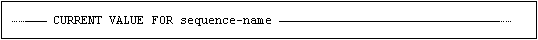Description

The result is the current value of the sequence specified in sequence-name. This is the value that was returned when the NEXT VALUE function was used for this sequence in this session.

This function can not be used until the initial value has been established for the sequence by using NEXT VALUE (i.e. using it immediately after the sequence has been created will raise an error).

The function can be used where a value-expression would normally be used. It can also be used after the DEFAULT clause in the CREATE DOMAIN, CREATE TABLE and ALTER TABLE statements.

USAGE privilege must be held on the sequence in order to use it.

Example

CREATE DOMAIN CHARGE_PERIOD_VALUE AS INTEGER

DEFAULT CURRENT VALUE FOR CHARGE_PERIOD_NO_SEQUENCE;

DAY

Returns the day of the month for the given date expression, expressed as an integer value in the range 1-31.

Syntax

Syntax for the DAY function: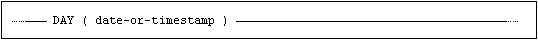date-or-timestamp is a date or timestamp value expression.

Description

The result is an integer value, 1 through 31.

If the value of date-or-timestamp is null, then the result of the function is null.

DAYOFMONTH

Returns the day of the month for the given date expression, expressed as an integer value in the range 1-31.

Syntax

Syntax for the DAYOFMONTH function: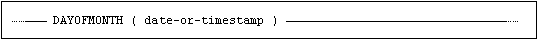date-or-timestamp is a date or timestamp value expression.

Description

The result is an integer value, 1 through 31.

If the value of date-or-timestamp is null, then the result of the function is null.

DAYOFWEEK

Returns the day of the week for the given date expression, expressed as an integer in the range 1-7.

Syntax

Syntax for the DAYOFYEAR function: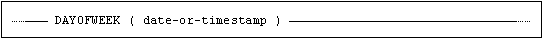date-or-timestamp is a date or timestamp value expression.

Description

The result is an integer value, 1 through 1, where 1 = Monday.

If the value of date-or-timestamp is null, then the result of the function is null.

Example

SET INT_VAL = DAYOFWEEK(CURRENT_DATE);  -- sets INT_VAL to the

-- day number of the current week

SET INT_VAL = DAYOFYEAR(DATE'2020-02-03');  -- sets INT_VAL to 1

DAYOFYEAR

Returns the day of the year for the given date expression, expressed as an integer in the range 1-366.

Syntax

Syntax for the DAYOFYEAR function: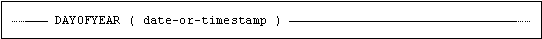date-or-timestamp is a date or timestamp value expression.

Description

The result is an integer value, 1 through 366, where 1 = January 1.

The value for a day after February 28 depends on whether the year is a leap year or not.

If the value of date-or-timestamp is null, then the result of the function is null.

Example

SET INT_VAL = DAYOFYEAR(CURRENT_DATE);  -- sets INT_VAL to the

-- day number of the current year

SET INT_VAL = DAYOFYEAR(DATE'2016-11-10');  -- sets INT_VAL to 315

SET INT_VAL = DAYOFYEAR(DATE'2017-11-10');  -- sets INT_VAL to 314

DEGREES

Returns an angle expressed in radians as degrees.

Syntax

Syntax for the DEGREES function: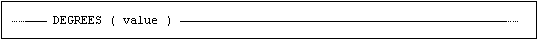value is a numeric value expression. The function handles values that are within the range of a double precision expression.

Rules

The function converts a numeric expression in radians to the corresponding values expressed in degrees. The data type for the result is double precision.

If the value of value is NULL, then the result of the function is NULL.

EXP

Returns the exponential value for a numeric expression.

Syntax

Syntax for the EXP function: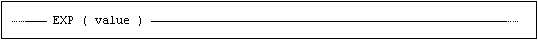value is a numeric value expression. The function handles values that are within the range of a double precision expression.

Rules

The function returns exponential value for the value expression. The data type for the result is double precision.

If the value of value is NULL, then the result of the function is NULL.

EXTRACT

Extracts a single field from a DATETIME or INTERVAL value.

Syntax

Syntax for the EXTRACT function: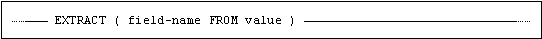Description

field-name is one of: YEAR, MONTH, DAY, HOUR, MINUTE or SECOND.

value must be of type DATETIME or INTERVAL and it must contain the field specified by field-name, otherwise an error is raised.

The data type of the result is integer.

The exception is when field-name is SECOND, in which case the result type is decimal where the precision is equal to the sum of the leading precision and the seconds precision of value, with a scale equal to the seconds precision.

When value is a negative INTERVAL, the result is a negative value. In all other cases the result is a positive value.

If the value of value is null, then the result of the function is null.

Example

SELECT CASE EXTRACT (MONTH FROM ARRIVE)

WHEN 1 THEN 'JANUARY'

WHEN 2 THEN 'FEBRUARY'

....

END

FROM TRAVELS

FLOOR

Returns the largest integer less than or equal to a numeric expression.

Syntax

Syntax for the FLOOR function: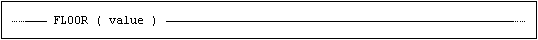value is a numeric value expression.

Description

The function returns the nearest integer value that is equal or lower to value.

If the value of value is null, then the result of the function is null.

The return data type is based on the input data type. For DECIMAL input, the return data type is integer.

Example

SET ? = FLOOR(13.13);    -- returns 13

SET ? = FLOOR(-13.13);   -- returns -14

SET ? = FLOOR(-12.34E1); -- returns -1.240000000E+002

HOUR

Returns the hour for the given time or timestamp expression, expressed as an integer value in the range 0-23.

Syntax

Syntax for the HOUR function: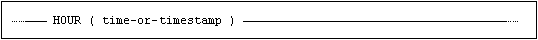time-or-timestamp is a time or timestamp value expression.

Description

The result is an integer value, 0 through 23, representing the hour.

If the value of time-or-timestamp is null, then the result of the function is null.

Example

SET H = HOUR(LOCALTIME);  -- sets H to the current hour number

INDEX_CHAR

Returns the index character for a string.

Syntax

Syntax for the INDEX_CHAR function: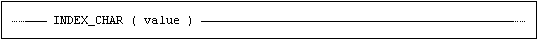value is a character value expression

Description

The result is a character value.

If the value of value is null, then the result of the function is null.

The INDEX_CHAR function takes a character string as argument and returns the index character for the string related to its collation. The default behavior is to return the first letter of the string, decomposed (accents removed) and capitalized (upper case).

However, many languages include accented letters, digraphs, and sometimes trigraphs as basic alphabetical characters. These combinations are properly handled by the INDEX_CHAR function.

Examples

SELECT INDEX_CHAR('östra aros' COLLATE english_1) FROM... -- will return 'O'

SELECT INDEX_CHAR('östra aros' COLLATE swedish_1) FROM... -- will return 'Ö'

IRAND

Returns a random integer number.

Syntax

Syntax for the IRAND function: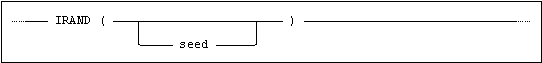seed is an integer value expression

Description

The result is a random integer value, in the range 0 to 2 147 483 647.

If a seed is given, this value is used to calculate the random value. If no seed is given, the value is calculated from the previous value. It is thus possible to generate the same random sequence by using the same seed.

Example

SET INT_VAL = MOD(IRAND(), 5);  -- sets INT_VAL to a random

-- value between 0 and 4

LEFT

Returns the specified number of leftmost characters in a given character string.

Syntax

Syntax for the LEFT function: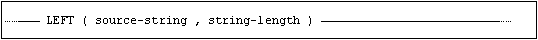source-string is a character or binary string expression.

string-length is an integer value expressions.

Description

The leftmost string-length characters of source-string are returned.

If count is zero, an empty string is returned.

If count is less than zero, then the result of the function is null.

If the value of either operand is null, then the result of the function is null.

Example

SET CHR_STR = LEFT('TEST STRING', 3);  -- sets CHR_STR to 'TES'

LN

Returns the natural logarithm for a numeric expression.

Syntax

Syntax for the LN function: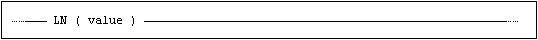value is a numeric value expression. The function handles values that are within the range of a double precision expression.

Rules

The function returns the natural logarithm for the value expression. The data type for the result is double precision. Valid input values are > 0.

If the value of value is NULL, then the result of the function is NULL.

LOCALTIME

Returns a TIME value denoting the current time (i.e. now).

Syntax

Syntax for the LOCALTIME function: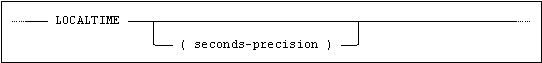seconds-precision is an unsigned integer value denoting the seconds precision for the returned TIME value.

Description

The result is the current time (i.e. now) as a TIME value.

The value of seconds-precision must be between 0 and 9.

If seconds-precision is not specified, the default value of 0 is assumed.

All references to LOCALTIME are effectively evaluated simultaneously from a single reading of the server clock. Thus the conditional expression LOCALTIME = LOCALTIME is guaranteed to always evaluate to true.

The value of LOCALTIME will always be equal to the TIME portion of LOCALTIMESTAMP.

Example

UPDATE EVENTS SET ADJUSTED = LOCALTIME   -- sets ADJUSTED to current time

WHERE ID = 81;                           -- (e.g. 15:45:02)

LOCALTIMESTAMP

Returns a TIMESTAMP denoting the current date and time.

Syntax

Syntax for the LOCALTIMESTAMP function: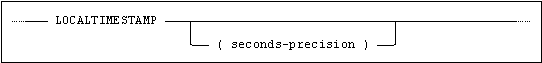seconds-precision is an unsigned integer value denoting the seconds precision for the returned TIMESTAMP value.

Description

The result is the current date and time as a TIMESTAMP value.

The value of seconds-precision must be between 0 and 9.

If seconds-precision is not specified, the default value of 6 is assumed.

All references to LOCALTIMESTAMP are effectively evaluated simultaneously from a single reading of the server clock. Thus the conditional expression LOCALTIMESTAMP = LOCALTIMESTAMP is guaranteed to always evaluate to true.

The value of LOCALTIMESTAMP will always be equal to the combined value of CURRENT_DATE and LOCALTIME.

Example

CREATE TABLE EVENTS(ID  INTEGER    PRIMARY KEY,

TS  TIMESTAMP  DEFAULT LOCALTIMESTAMP);

INSERT INTO EVENTS(ID) VALUES (1);  -- default value for TS inserted

-- (e.g. 2019-09-27 16:14:07.230000)

UPDATE EVENTS

SET    TS = LOCALTIMESTAMP

WHERE  ID <= 10;

LOCATE

Returns the starting position of the first occurrence of a specified string expression in a given character string, starting from an optional start position, or the left of the character string.

Syntax

Syntax for the LOCATE function: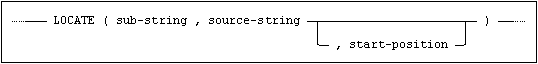sub-string and source-string are character or binary string expressions.

start-position is an integer value expression.

Description

The position of the first occurrence of sub-string in source-string is returned, starting from the position specified by start-position if given, otherwise from position 1, in source-string (the left-most position).

If sub-string does not occur in source-string, the functions returns zero.

If the length of source-string is zero, the function returns zero.

If the length of source-string is less than start-position, the function returns zero.

If the length of sub-string is zero, the function returns 1.

If the value of any operand is null, then the result of the function is null.

Example

SET INT_VAL = LOCATE('NA', 'BANANA', 4); -- sets INT_VAL to 5

LOG10

Returns the base-10 logarithm for a numeric expression.

Syntax

Syntax for the LOG10 function: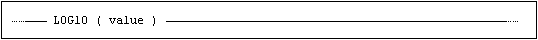value is a numeric value expression. The function handles values that are within the range of a double precision expression.

Rules

The function returns the base-10 logarithm for the value expression. The data type for the result is double precision. Valid input values are > 0.

If the value of value is NULL, then the result of the function is NULL.

LOWER

Converts all uppercase letters in a character string to lowercase.

Syntax

Syntax for the LOWER function: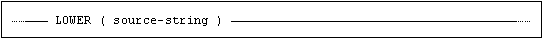source-string is a character string expression.

Description

The data type of the result is the same as the data type of source-string.

source-string is either in character or national character (i.e. Unicode) format.

If the value of source-string is null, then the result of the function is null.

Note:The length of the result may be longer or shorter than the input value. This means that using LOWER (or UPPER) on a column may cause data truncation.

Example

SELECT CHAR_LENGTH(TRIM(DESCRIPTION)), LOWER(TRIM(DESCRIPTION))

FROM   CHARGES;

MINUTE

Returns the minute for the given time or timestamp expression, expressed as an integer value in the range 0-59.

Syntax

Syntax for the MINUTE function: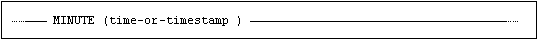time-or-timestamp is a time or timestamp value expression.

Description

The result is an integer value, 0 through 59, representing the minute.

If the value of time-or-timestamp is null, then the result of the function is null.

Example

SET M = MINUTE(LOCALTIME);  -- sets M to the current minute number

MOD

Returns the remainder (modulus) of a specified integer expression divided by a second specified integer expression.

Syntax

Syntax for the MOD function: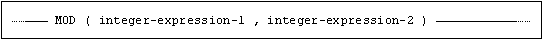integer-expression-1 and integer-expression-2 are integer value expressions.

Description

The result is the remainder of integer-expression-1 divided by integer-expression-2.

If the value of integer-expression-2 is zero, a divide-by-zero error will be raised.

The sign of the result is the same as the sign of integer-expression-1.

If the value of either operand is null, then the result of the function is null.

Note:Mimer SQL also supports the non-standard % modulo operator.

Example

SET INT_VAL = MOD(IRAND(), 5);  -- sets INT_VAL to a random

-- value between 0 and 4

SET INT_VAL = IRAND() % 5;      -- sets INT_VAL to a random

-- value between 0 and 4

MONTH

Returns the month for the given date or timestamp expression, expressed as an integer value in the range 1-12.

Syntax

Syntax for the MONTH function: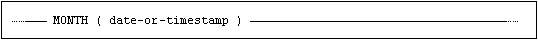date-or-timestamp is a date or timestamp value expression.

Description

The result is an integer value, 1 through 12, representing the month.

If the value of date-or-timestamp is null, then the result of the function is null.

Example

SET M = MONTH(CURRENT_DATE);  -- sets M to the current month number

NEXT VALUE

Returns the next value in the series of values defined by a sequence, provided that the last value in that series has not already been reached.

Syntax

Syntax for the NEXT VALUE function: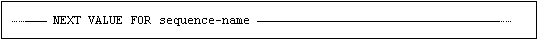Description

The result will be the next value in the series of the values defined by the sequence specified in sequence-name (this value will then become the session’ current value for the sequence).

If the sequence is unique (i.e. NO CYCLE option) and the current value of the sequence specified in sequence-name is already equal to the last value in the series of the values defined by it an error will be raised and the current value of the sequence will remain unchanged.

If the sequence is non-unique, the function will always succeed. If the current value of the sequence specified in sequence-name is equal to the last value in the series of values generated by the sequence, the initial value of the sequence will be returned.

The function can be used where a value-expression would normally be used. It can also be used after the DEFAULT clause in the CREATE DOMAIN, CREATE TABLE and ALTER TABLE statements.

This function is used to establish the initial value of the sequence after it has been created using the CREATE SEQUENCE statement.

USAGE privilege must be held on the sequence in order to use it.

Note:If the NEXT VALUE function is used in a select clause the sequence will be incremented for each row returned by the query.

Example

SET Z = NEXT VALUE FOR Z_SEQUENCE;

OCTET_LENGTH

Returns the octet (byte) length of a string. For single-octet character sets this is the same as CHARACTER_LENGTH.

Syntax

Syntax for the OCTET_LENGTH function: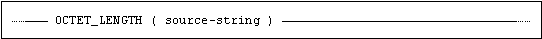source-string is a character or binary string expression.

Description

OCTET_LENGTH returns an INTEGER value.

If the data type of source-string is variable-length character or variable length binary, then the result of OCTET_LENGTH is the same as the actual length of source-string in octets.

If the data type of source-string is fixed-length character or fixed-length binary, then the result of OCTET_LENGTH is the same as the fixed-length of source-string.

If the data type of source-string is variable-length national character, then the result of OCTET_LENGTH is the same as the actual length of source-string in octets, i.e. 4 times the actual number of characters.

If the data type of source-string is fixed-length national character, then the result of OCTET_LENGTH is the same as 4 times the fixed-length of source-string.

If the value of source-string is null, then the result of the function is null.

Example

SET INT_VAL = OCTET_LENGTH(X'4142');  -- sets INT_VAL to 2

SET INT_VAL = OCTET_LENGTH('ABC');    -- sets INT_VAL to 3

SET INT_VAL = OCTET_LENGTH(n'ABC');   -- sets INT_VAL to 12

OVERLAY

Returns a character string where a number of characters, beginning at a given position, have been deleted from a character string and replaced with a given string expression.

Syntax

Syntax for the OVERLAY function: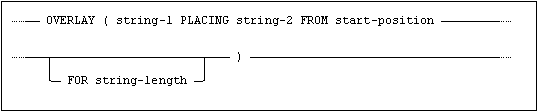string-1 and string-2 are character or binary string expressions.

string-1 and string-2 must be of the same type, i.e. either both character or both binary.

start-position and string-length are integer value expressions.

Description

The string-length number of characters in string-1, starting from position start-position are deleted from string-1. Then string-2 is inserted into string-1, at the ‘point of deletion’. The resulting character or binary string is returned.

If the value of string-length is positive, the string-length number of characters to the right of start-position are deleted. If the value of string-length is negative, the string-length number of characters to the left of start-position are deleted.

The point-of-deletion is where the cursor would be if you had just used a text editor to select the characters, as described, and performed an edit-cut operation.

A value for start-position of less than 1 (zero or negative) specifies a position to the left of the beginning of string-1.

It is possible that the specified deletion may not actually affect any of the characters of string-1, in which case the OVERLAY operation produces the effect of a prepend.

If the value of any operand is null, then the result of the function is null.

string-2 must not contain Unicode characters outside the Latin1 repertoire if string-1 is of character type.

Example

OVERLAY('ABCDEF' PLACING 'ab' FROM 2 FOR 3);  -- returns 'AabEF'

OVERLAY('ABCDEF' PLACING 'ab' FROM 2);        -- returns 'AabDEF'

PASTE

Returns a character string where a specified number of characters, beginning at a given position, have been deleted from a character string and replaced with a given string expression.

Syntax

Syntax for the PASTE function: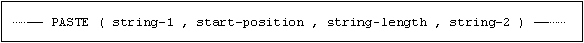string-1 and string-2 are character or binary string expressions.

string-1 and string-2 must be of the same type, i.e. either both character or both binary.

start-position and string-length are integer value expressions.

Description

The string-length number of characters in string-1, starting from position start-position are deleted from string-1. Then string-2 is inserted into string-1, at the ‘point of deletion’. The resulting character or binary string is returned.

If the value of string-length is positive, the string-length number of characters to the right of start-position are deleted. If the value of string-length is negative, the string-length number of characters to the left of start-position are deleted.

The point-of-deletion is where the cursor would be if you had just used a text editor to select the characters, as described, and performed an edit-cut operation.

A value for start-position of less than 1 (zero or negative) specifies a position to the left of the beginning of string-1.

It is possible that the specified deletion may not actually affect any of the characters of string-1, in which case the PASTE operation produces the effect of a prepend.

If the value of any operand is null, then the result of the function is null.

string-2 must not contain Unicode characters outside the Latin1 repertoire if string-1 is of character type.

Example

SET CHR_STR = PASTE('TEST STRING', 6, 3, 'P');  -- sets CHR_STR to 'TEST PING'

POSITION

Returns the starting position of the first occurrence of a specified string expression in a given character string, starting from the left of the character string.

Syntax

Syntax for the POSITION function: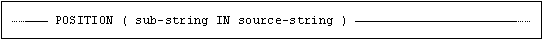sub-string and source-string are character or binary string expressions.

sub-string and source-string must be of the same type, i.e. either both character or both binary.

Description

The position of the first occurrence of sub-string in source-string is returned, starting from position 1 in source-string (the left-most position).

If sub-string does not occur in source-string, the functions returns zero.

If the length of source-string is zero, the function returns zero.

If the length of sub-string is zero, the function returns 1.

If the value of either operand is null, then the result of the function is null.

Example

SET INT_VAL = POSITION('STR' IN 'TEST STRING'); -- sets INT_VAL to 6

POWER

Returns the specified numeric expression, raised to the power of the given value.

Syntax

Syntax for the POWER function: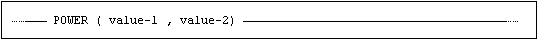value is a numeric value expression. The function handles values that are within the range of a double precision expression.

Rules

The function returns the value of the first argument raised to the power of the second argument. The data type for the result is double precision.

If the value of value-1 or value-2 is NULL, then the result of the function is NULL.

QUARTER

Returns the quarter for the given date or timestamp expression, expressed as an integer value in the range 1-4.

Syntax

Syntax for the QUARTER function: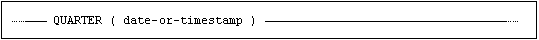date-or-timestamp is a date or timestamp value expression.

Description

The result is an integer value, 1 through 4, representing the quarter.

If the value of date-or-timestamp is null, then the result of the function is null.

Example

SET Q = QUARTER(CURRENT_DATE);  -- sets Q to the current quarter number

Returns an angle expressed in degrees as radians.

Syntax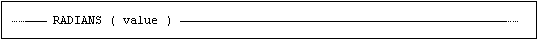value is a numeric value expression. The function handles values that are within the range of a double precision expression.

Rules

The function converts a value expressed in degrees to the corresponding value expressed as radians. The data type for the result is double precision.

If the value of value is NULL, then the result of the function is NULL.

REGEXP_MATCH

Performs a regular expression comparison.

Syntax

Syntax for the REGEXP_MATCH function: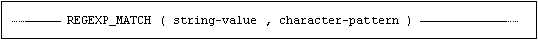Description

The REGEXP_MATCH function compares the value in a string expression with a character string pattern which may contain different meta-characters.

Compared to LIKE, the regular expression provides a much more flexible way to match strings of text, such as complex patterns of characters.

Regular expression constructs

Characters

x

The character x

\

Escape for meta characters: \$ & ( ) * + , - . ? [ ] ^ { | }

\\

The backslash character

\t

The tab character

\n

The newline character

\v

The vertical tab character

\f

The form feed character

\r

The carriage return character

\x{h...h}

The character with hex value 0xh...h (<= 0x10FFFF)

Character classes

[abc]

a, b, or c (simple class)

[^abc]

Any character except a, b, or c (negation)

[a-zA-Z]

a through z or A through Z, inclusive (range)

[[a-d][m-p]]

a through d, or m through p (union)

[[a-z]&&[def]]

d, e, or f (intersect)

[[a-z]--[bc]]

a through z, except for b and c (minus)

Predefined character classes

.

Any character

\d

A digit character

\D

Not a digit character ([^\d])

\s

A whitespace character

\S

Not a whitespace character ([^\s])

\w

A word character

\W

Not a word character ([^\w])

Boundary matchers

^

The beginning of string

\$

The end of string

Quantifiers

X?

X, once or not at all

X*

X, zero or more times

X+

X, one or more times

X{n}

X, exactly n times

X{n,}

X, at least n times

X{n,m}

X, at least n but not more than m times

Logical operators

XY

X followed by Y

X|Y

Either X or Y

(X)

X, as a capturing group

Classes for Unicode categories

\p{L}

Letter

\p{Ll}

Lowercase_Letter

\p{Lu}

Uppercase_Letter

\p{Lt}

Titlecase_Letter

\p{Lm}

Modifier_Letter

\p{Lo}

Other_Letter

\p{N}

Number

\p{Nd}

Decimal_Digit_Number

\p{Nl}

Letter_Number

\p{No}

Other_Number

\p{M}

Mark

\p{Mn}

Non_Spacing_Mark

\p{Mc}

Spacing_Combining_Mark

\p{Me}

Enclosing_Mark

\p{P}

Punctuation

\p{Pd}

Dash_Punctuation

\p{Ps}

Open_Punctuation

\p{Pe}

Close_Punctuation

\p{Pi}

Initial_Punctuation

\p{Pf}

Final_Punctuation

\p{Pc}

Connector_Punctuation

\p{Po}

Other_Punctuation

\p{S}

Symbol

\p{Sm}

Math_Symbol

\p{Sc}

Currency_Symbol

\p{Sk}

Modifier_Symbol

\p{So}

Other_Symbol

\p{Z}

Separator

\p{Zs}

Space_Separator

\p{Zl}

Line_Separator

\p{Zp}

Paragraph_Separator

\p{C}

Other

\p{Cc}

Control

\p{Cf}

Format

\p{Co}

Private_Use

\p{Cn}

Unassigned

Classes for Unicode scripts

\p{Arabic}

\p{Armenian}

\p{Katakana}

\p{Bengali}

\p{Khmer}

\p{Bopomofo}

\p{Lao}

\p{Cherokee}

\p{Latin}

\p{Common}

\p{Malayalam}

\p{Cyrillic}

\p{Mongolian}

\p{Devanagari}

\p{Myanmar}

\p{Ethiopic}

\p{Oriya}

\p{Georgian}

\p{Sinhala}

\p{Greek}

\p{Syriac}

\p{Gujarati}

\p{Tamil}

\p{Gurmukhi}

\p{Telugu}

\p{Han}

\p{Thaana}

\p{Hangul}

\p{Thai}

\p{Hebrew}

\p{Tibetan}

\p{Hiragana}

\p{Yi}

Examples

regexp_match(search_string,'abc')

The regexp_match function will return TRUE if the search_string anywhere has the sequence abc. Note the difference with the like predicate where the same criteria would need to be expressed as

search_string like '%abc%'

Escape of meta characters are done using a backslash character:

regexp_match(search_string,'\[abc\]')

would be true if search_string anywhere contains the string [abc], (including the square brackets).

By using the boundary characters ^ and \$ it is possible to specify that a search string should start with or end with some specific characters. E.g.

regexp_match(search_string,'^Mimer')

would return true if the search_string started with the letters Mimer. For this type of searches, the database will consider using an index if appropriate.

The regexp_match function is collation aware. Thus

regexp_match('AAlborg' collate danish_1,'ålborg')

is true while

regexp_match('AAlborg' collate danish_2,'ålborg')

is false since a collation for danish will match AA to Å, but the level 1 collation is case insensitive which the level 2 collation is not.

This far, all of the examples given, can also be expressed with the like predicate. The following examples will deal with ranges and quantifiers which can be used to specify more complex search patterns.

To search for non-printable characters the regular expression

'[\x{0}-\x{1B}]'

could be used.

To find strings beginning with An or A, regardless of case, followed by a space and one or more arbitrary characters the pattern would be

'^(A|an)|A|n .+'

The pattern

'[a-zA-Z]{3}.[0-9]{3}'

would match a string containing three occurrences of a letter between a and z or A and Z, followed by an arbitrary character and three consecutive digits.

General information about the different classes for Unicode categories can be found at https://www.unicode.org/reports/tr18/ and https://www.unicode.org/reports/tr44/. Please note that these documents cover lots of functionality not supported by Mimer SQL.

REPEAT

Returns a character string composed of a specified string expression repeated a given number of times.

Syntax

Syntax for the REPEAT function: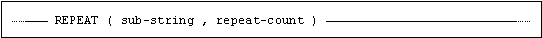sub-string is a character or binary string expression.

repeat-count is an integer expression.

Description

The result is a character or binary string consisting of sub-string repeated repeat-count times.

If the value of repeat-count is zero, then the result of the function is a character or binary string of length zero.

If the value of repeat-count is less than zero, then the result of the function is null.

If the value of either operand is null, then the result of the function is null.

Example

SET CHR_STR = REPEAT('ABC', 3);  -- sets CHR_STR to 'ABCABCABC'

REPLACE

Replaces all occurrences of a given string expression with another string expression in a character string.

Syntax

Syntax for the REPLACE function: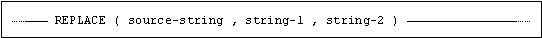source-string, string-1 and string-2 are character or binary string expressions.

source-string, string-1 and string-2 must be of equal type, i.e. either all are character or all are binary.

Description

All occurrences of string-1 found in source-string are replaced with string-2, the resulting character or binary string is returned.

If the value of any of the operands is null, then the result of the function is null.

string-2 must not contain Unicode characters outside the Latin1 repertoire if source-string is of character type.

Example

SET CHR_STR = REPLACE('TEST STRING', 'ST', 'NOR');  -- sets CHR_STR to

-- 'TENOR NORRING'

RIGHT

Returns the specified number of rightmost characters in a given character string.

Syntax

Syntax for the RIGHT function: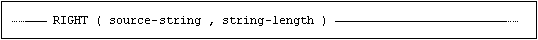source-string is a character or binary string expression.

string-length is an integer value expressions.

Description

The rightmost string-length characters of source-string are returned.

If count is zero, an empty string is returned.

If count is less than zero, then the result of the function is null.

If the value of either operand is null, then the result of the function is null.

Example

SET CHR_STR = RIGHT('TEST STRING', 3);  -- sets CHR_STR to 'ING'

ROUND

Rounds a numeric value.

Syntax

Syntax for the ROUND function: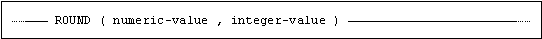numeric-value is an integer or a float value expression.

integer-value is an integer value expression.

Description

If integer-value is positive, the value describes the number of digits permitted in numeric-value, after rounding, to the right of the decimal point, if it is negative it describes the number of digits allowed to the left of the decimal point.

The value returned depends on the data type of numeric-value.

If the value of either operand is null, then the result of the function is null.

Returns the given numeric expression rounded to the number of places to the right of the decimal point specified by a given integer expression.

If the integer expression is negative, the numeric expression is rounded to a number of places to the left of the decimal point specified by the absolute value of the integer expression.

Examples

SET :NUM_VAL = ROUND(762.847, 2);   -- sets NUM_VAL to 762.850

SET :NUM_VAL = ROUND(762.847, 1);   -- sets NUM_VAL to 762.800

SET :NUM_VAL = ROUND(762.847, 0);   -- sets NUM_VAL to 763.000

SET :NUM_VAL = ROUND(762.847, -1);  -- sets NUM_VAL to 760.000

SET :NUM_VAL = ROUND(762.847, -2);  -- sets NUM_VAL to 800.000

SET :NUM_VAL = ROUND(7654, -2);     -- sets NUM_VAL to 7700

SECOND

Returns the second for the given time or timestamp expression, expressed as an integer value in the range 0-59.

Syntax

Syntax for the SECOND function: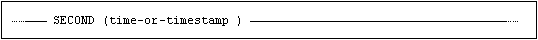time-or-timestamp is a time or timestamp value expression.

Description

The result is an integer value, 0 through 59, representing the second.

If the value of time-or-timestamp is null, then the result of the function is null.

Example

SET INT_VAL = SECOND(LOCALTIMESTAMP);  -- sets INT_VAL to the second number

SESSION_USER

Returns the name of the currently connected ident.

Syntax

Syntax for the SESSION_USER function: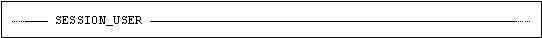Description

The result is the name of the current ident (i.e. the ident who established the current database connection).

The data type of the returned value is nchar varying with a maximum length of 128, with the collation SQL_IDENTIFIER.

Example

The following example returns the Program ident if entered, otherwise the session ident:

SET CHR_STR = COALESCE(CURRENT_PROGRAM(), SESSION_USER);

SIGN

Returns an indicator of the sign of the given numeric expression.

If the numeric expression is less than zero, -1 is returned. If the numeric expression is equal to zero, 0 is returned. If the numeric expression is greater than zero, 1 is returned.

Syntax

Syntax for the SIGN function: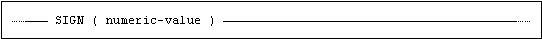numeric-value is an integer or a float value expression.

Description

The function returns an indicator of the sign of numeric-value. If numeric-value is less than zero, -1 is returned. If numeric-value equals zero, 0 is returned. If numeric-value is greater than zero, 1 is returned.

If the value of numeric-value is null, then the result of the function is null.

Examples

SET INT_VAL = SIGN(-12);  -- sets INT_VAL to -1

SET INT_VAL = SIGN(0);    -- sets INT_VAL to 0

SET INT_VAL = SIGN(12);   -- sets INT_VAL to 1

SIN

Returns the sine for a numeric expression.

Syntax

Syntax for the SIN function: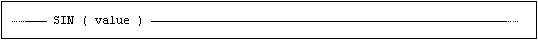value is a numeric value expression. The function handles values that are within the range of a double precision expression.

Rules

The function returns the sine for the value expressed as radians. The data type for the result is double precision.

If the value of value is NULL, then the result of the function is NULL.

SINH

Returns the hyperbolic sine for a numeric expression.

Syntax

Syntax for the SINH function: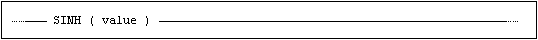value is a numeric value expression. The function handles values that are within the range of a double precision expression.

Rules

The function returns the hyperbolic sine for the value expressed as radians. The data type for the result is double precision.

If the value of value is NULL, then the result of the function is NULL.

SOUNDEX

Returns a character string value containing six digits that represent an encoding of the sound of the given string expression.

Syntax

Syntax for the SOUNDEX function: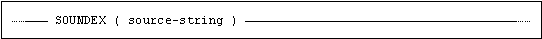source-string is a character string expression.

Description

The function returns a character string value containing six digits that represent an encoding of the sound of source-string.

If source-string contains two or more words, they are effectively concatenated into a single word by ignoring the separating space characters.

If the SOUNDEX values for two strings compare to be equal then they sound the same.

If the value of source-string is null, then the result of the function is null.

SQRT

Returns the square root of a numeric expression.

Syntax

Syntax for the SQRT function: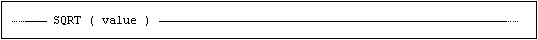value is a numeric value expression. The function handles values that are within the range of a double precision expression.

Rules

The function returns the square root of the value. The data type for the result is double precision. Valid input values are greater than or equal to 0.

If the value of value is NULL, then the result of the function is NULL.

SUBSTRING

Extracts a substring from a given string, according to specified start position and length of the substring.

Syntax

Syntax for the SUBSTRING function: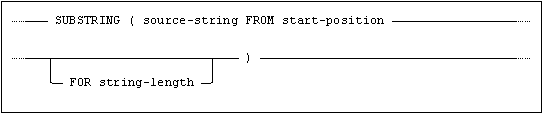source-string is a character or binary string expression.

start-position and string-length are integer value expressions.

Alternative, comma separated syntax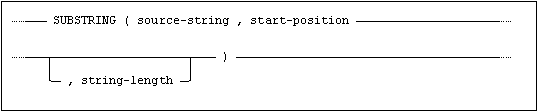Description

SUBSTRING returns a character or binary string containing string-length characters of source-string, starting at the character specified by start-position, and in the same sequence as they appear in source-string.

If any of these positions are before the start or after the end of source-string, then no character is returned for that position. If all positions are outside the source string, an empty string is returned.

The first character in source-string has position 1.

If the data type of source-string is variable-length character, then the result of the SUBSTRING function is a variable-length character with maximum string length equal to the maximum length of source-string. If the data type of source-string is fixed-length character, then the result of the SUBSTRING function is a variable-length character with maximum string length equal to the fixed length of source-string.

If the data type of source-string is variable-length binary, then the result of the SUBSTRING function is a variable-length binary with maximum string length equal to the maximum length of source-string. If the data type of source-string is fixed-length binary, then the result of the SUBSTRING function is a variable-length binary with maximum string length equal to the fixed length of source-string.

If string-length is negative, or if start-position is greater than the number of characters in source-string, the function fails and an error is returned.

If string-length is omitted then it is assumed to be:

CHAR_LENGTH(source-string) + 1 - start-position

i.e. the remainder of source-string, starting at start-position, is returned.

If the value of any operand is null, then the result of the function is null.

Character strings returned from a SUBSTRING function, inherit the collation from the source string.

Example

SET CHR_STR = SUBSTRING('Whatever' FROM 3 FOR 3); -- sets CHR_STR to 'ate'

TAIL

Returns the specified number of rightmost characters in a given character string.

Syntax

Syntax for the TAIL function: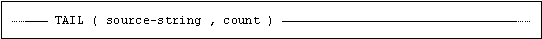source-string is a character or binary string expression.

count is an integer value expression.

Description

The rightmost count characters of source-string are returned.

If count is zero, an empty string is returned.

If count is less than zero, then the result of the function is null.

If the value of either operand is null, then the result of the function is null.

Example

SET CHR_STR = TAIL('TEST STRING', 3);  -- sets CHR_STR to 'ING'

TAN

Returns the tangent for a numeric expression.

Syntax

Syntax for the TAN function: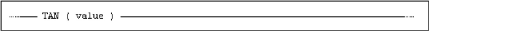value is a numeric value expression. The function handles values that are within the range of a double precision expression.

Rules

The function returns the tangent for the value expressed as radians. The data type for the result is double precision.

If the value of value is NULL, then the result of the function is NULL.

TANH

Returns the hyperbolic tangent for a numeric expression.

Syntax

Syntax for the TANH function: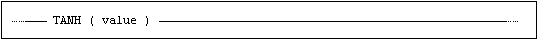value is a numeric value expression. The function handles values that are within the range of a double precision expression.

Rules

The function returns the hyperbolic tangent for the value expressed as radians. The data type for the result is double precision.

If the value of value is NULL, then the result of the function is NULL.

TRIM

Removes leading and/or trailing instances of a specified character from a string.

Syntax

Syntax for the TRIM function: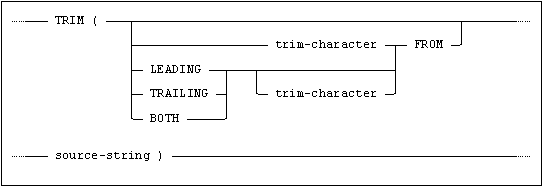trim-character is a character or binary string expression of length 1.

source-string is a character or binary string expression.

source-string and trim-character must be of equal type, i.e. either must both be character or both binary.

Note:LEADING, TRAILING or BOTH is referred to as the trim-specification below.

Description

If trim-character is not specified, ' ' (space) is implicit for character data, and x'00' is implicit for binary data.

If trim-specification is not specified, BOTH is implicit.

If the data type of source-string is variable-length character, then the result of the TRIM function is a variable-length character with maximum string length equal to the maximum length of source-string. If the data type of source-string is fixed-length character, then the result of the TRIM function is a variable-length character with maximum string length equal to the length of source-string.

If the data type of source-string is variable-length binary, then the result of the TRIM function is a variable-length binary with maximum string length equal to the maximum length of source-string. If the data type of source-string is fixed-length binary, then the result of the TRIM function is a variable-length binary with maximum string length equal to the length of source-string.

If the length of trim-character is not 1, an error is returned.

If the value of either operand is null, then the result of the function is null.

Character strings returned from a TRIM function, inherit the collation from the source string.

Examples

SET CHR_STR = TRIM(' TEST ');                  -- sets CHR_STR to 'TEST'

SET CHR_STR = TRIM('T' FROM 'TEST');           -- sets CHR_STR to 'ES'

SET CHR_STR = TRIM(LEADING 'T' FROM 'TEST');   -- sets CHR_STR to 'EST'

SET CHR_STR = TRIM(TRAILING 'T' FROM 'TEST');  -- sets CHR_STR to 'TES'

TRUNCATE

Returns the given numeric expression truncated to a number of places to the right of the decimal point specified by a given integer expression.

If the integer expression is negative, the numeric expression is truncated to a number of places to the left of the decimal point specified by the absolute value of the integer expression.

Syntax

Syntax for the TRUNCATE function: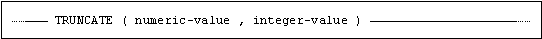numeric-value is an integer or a float value expression.

integer-value is an integer value expression.

Description

If integer-value is positive, the value describes the number of digits permitted in numeric-value, after truncation, to the right of the decimal point.

If it is negative, it describes the number of digits allowed to the left of the decimal point.

The value returned depends on the data type of numeric-value.

If the value of either operand is null, then the result of the function is null.

Examples

SET NUM_VAL = TRUNCATE(25.89, 1);   -- sets NUM_VAL to 25.80

SET NUM_VAL = TRUNCATE(25.89, -1);  -- sets NUM_VAL to 20.00

UNICODE_CHAR

Returns the character that has the given Unicode scalar value.

Syntax

Syntax for the UNICODE_CHAR function: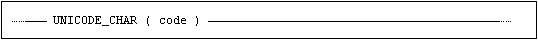code is a numeric expression representing a Unicode scalar value.

Description

If the value of code represents a valid Unicode character, the function returns a single national character value, i.e. NCHAR(1), otherwise an error is raised.

If the value of code is null, then the result of the function is null.

Example

SET NCHR_VAL = UNICODE_CHAR(65);  -- sets NCHR_VAL to 'A'

UNICODE_CODE

Returns the Unicode scalar value of the leftmost character in the given string expression, as an integer.

Syntax

Syntax for the UNICODE_CODE function: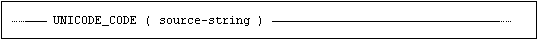source-string is a character or binary string expression.

Description

A single INTEGER value is returned, representing a Unicode scalar value.

If the source-string contains more than one character, the Unicode scalar value of the left-most character is returned.

If the length of source-string is zero, then the result of the function is null.

If the value of source-string is null, then the result of the function is null.

Example

SET INT_VAL = UNICODE_CODE(n'A'); -- sets INT_VAL to 65

UPPER

Converts all lowercase letters in a character string to uppercase.

Syntax

Syntax for the UPPER function: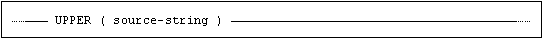source-string is a character string expression.

Description

The data type of the result is the same as the data type of source-string.

source-string is either in character or national character (i.e. Unicode) format.

If the value of source-string is null, then the result of the function is null.

Note:The length of a result may be longer or shorter than the input value. This means that using UPPER on a column may cause data truncation.

USER

Returns the same value as CURRENT_USER. We recommend that you use CURRENT_USER, see CURRENT_USER.

WEEK

Returns the week of the year for the given date or timestamp expression, expressed as an integer value in the range 1-53.

Syntax

Syntax for the WEEK function: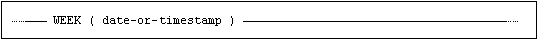date-or-timestamp is a date or timestamp value expression.

Description

The result is an integer value, 1 through 53, representing the week number in the year, calculated in accordance with the ISO 8601 standard. (The year’s first week with 4 or more days is week 1.)

If the value of date-or-timestamp is null, then the result of the function is null.

Example

SET INT_VAL = WEEK(CURRENT_DATE);  -- sets INT_VAL to the week number

-- of the current year

YEAR

Returns the year for the given date or timestamp expression, expressed as an integer value in the range 1-9999.

Syntax

Syntax for the YEAR function: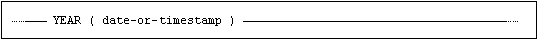date-or-timestamp is a date or timestamp value expression.

Description

The result is an integer value, 1 through 9999, representing the year.

If the value of date-or-timestamp is null, then the result of the function is null.

Example

SET INT_VAL = YEAR(CURRENT_DATE);  -- sets INT_VAL to the year number

-- of the current year

Standard Compliance

This section summarizes standard compliance for scalar functions.

Standard

Compliance

SQL-2016

Core

Fully compliant.

SQL-2016

Features outside core

Feature F052, “Intervals and datetime arithmetic”.

Feature F555, “Enhanced seconds precision” LOCALTIME and LOCALTIMESTAMP functions with fractions of seconds.

Feature T176, “Sequence generator support”.

Feature T441, “Support for ABS and MOD functions”.

Feature T621, “Enhanced numeric functions”.

Feature T622, “Trigonometric functions”.

Feature T624, “Common logarithm functions”.

Mimer SQL extension

Support for:
ASCII_CHAR
ASCII_CODE
ATAN2
BEGINS
BUILTIN.BEGINS_WORD
BUILTIN.MATCH_WORD
BUILTIN.UTC_TIMESTAMP
COT
CURRENT_PROGRAM
CURRENT VALUE
DAYOFMONTH
DAYOFWEEK
DAYOFYEAR
DEGREES
HOUR
INDEX_CHAR
IRAND
LEFT
LOCATE
MONTH
PASTE
QUARTER
REGEXP_MATCH
REPEAT
REPLACE
ROUND
SECOND
SIGN
SOUNDEX
TAIL
TRUNCATE
UNICODE_CHAR
UNICODE_CODE
WEEK
YEAR

is a Mimer SQL extension.

Set Functions

Set functions are pre-defined functions used in select specifications. They operate on the set of values in one column of the result of the SELECT statement, or on the subset in a group if the statement includes a GROUP BY clause.

The result of a set function is a single value for each operand set.

Syntax for Set Functions

The general syntax for a set function is: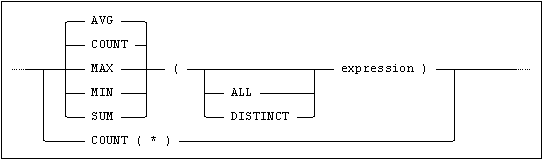AVG

Returns the average of the values in the set.

Note:AVG can only be applied to numerical and interval values.

COUNT

Returns the number of values in the set.

MAX

Returns the largest value in the set.

MIN

Returns the smallest value in the set.

SUM

Returns the sum of the values in the set.

Note:SUM can only be applied to numerical and interval values.

Examples

SELECT MIN(PRICE) AS INEXPENSIVE, MAX(PRICE) AS EXPENSIVE

FROM   ROOM_PRICES WHERE HOTELCODE = 'LAP';

SELECT HOTELCODE, AVG(PRICE) AS AVERAGE_PRICE

FROM   ROOM_PRICES

GROUP BY HOTELCODE;

SELECT COUNT(*) FROM SOME_TABLE;

Operational Mode

The operational mode of a set function is determined by the use of the keywords ALL and DISTINCT.

When ALL is specified or no keyword is used:

Any duplicate values in the operand set are retained.

When DISTINCT is specified:

Redundant duplicate values are eliminated from the operand set before the function is applied.

The result of the set function must not be combined with other terms using binary arithmetic operators.

For the set functions MAX and MIN, the DISTINCT keyword makes no difference to the result. (The same value will be returned with or without DISTINCT.)

Null Values

For all set functions except COUNT(*), any null values in the operand set are eliminated before the set function is applied, regardless of whether DISTINCT is specified or not.

The special form COUNT(*) returns the number of rows in the result table, including any null values. The keywords ALL and DISTINCT may not be used with this form of COUNT.

If the operand set is empty, the COUNT function returns the value zero. All other functions return null for an empty operand set.

The COUNT function returns an INTEGER. The MAX and MIN functions return a value with the same type and precision as the operand. The precision of the result returned by SUM and AVG is considered below.

Restrictions

Column references in the argument of a set function may not address view columns which are themselves derived from set functions.

The argument of a set function must contain at least one column reference and cannot contain any set function references. If the column is an outer reference, then the expression should not include any operators.

If a set function contains a column that is an outer reference, then the set function must be contained in a subquery of a HAVING clause.

Results of Set Functions

When the argument of a set function is a numerical value, the precision and scale of the set function result is evaluated in accordance with the rules given below. If the argument is an expression, the expression is first evaluated as described in Expressions before the set function is applied.

Evaluating Set Functions

 FLOAT(p') INTEGER(p') DECIMAL(p',s') SUM FLOAT(p)a INTEGER(p)b DECIMAL(p,s)c AVG FLOAT(p)a INTEGER(p)d DECIMAL(p,s)e MAX, MIN FLOAT(p)d INTEGER(p)d DECIMAL(p,s)f COUNT INTEGER(10) INTEGER(10) INTEGER(10)
 ap=max(15, p’) bp=min(45, 10+p’) cp=min(45, 10+p') s=s' dp=p’ ep=min(45, 10+p') s=p-(p'-s') fp=p' s=s'

The following examples show how some set functions are evaluated.

AVG(SMALLINT) gives SMALLINT

AVG(INTEGER) gives INTEGER

AVG(DECIMAL(38,10)) gives DECIMAL(45,17)

AVG(DECIMAL(4,2)) gives DECIMAL(14,12)

AVG(INTERVAL YEAR(2) TO MONTH) gives INTERVAL YEAR(2) TO MONTH

SUM(SMALLINT) gives INTEGER(15)

SUM(INTEGER) gives INTEGER(20)

SUM(DECIMAL(38,10)) gives DECIMAL(45,10)

SUM(DECIMAL(4,2)) gives DECIMAL(14,2)

SUM(INTERVAL YEAR(2) TO MONTH) gives INTERVAL YEAR(2) TO MONTH

Note:Often, the average of a series of integers is required as a decimal rather than an integer. This may be achieved by casting the value to a decimal using the CAST function.
For example, if the values in the integer column COL are 1, 3 and 6, then AVG(COL) returns 3 but AVG(CAST(COL as decimal(14,4))) returns 3.33333333333333.

Alternatively, multiply the AVG argument by 1.0, i.e. AVG(COL * 1.0).

Standard Compliance

This section summarizes standard compliance for set functions.

Standard

Compliance

SQL-2016

Core

Fully compliant.

SQL-2016

Features outside core

Feature F441, “Extended set function support”.

Feature F561, “Full value expressions” use of DISTINCT expression in set function, where expression is not a column.